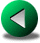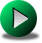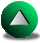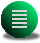Previous: Finite Difference Models
Next: The Discontinuous Spectral Element
Up: Presentation of the Numerical

Subsections

# Finite Element Models

## Introduction

In this section, we present several finite element (FE) models, all based on triangular elements. The development of the FE method was contiguous to the development of computers in the early 60s and 70s. By the end of 70s, they were well established. They became particularly popular in engineering for the computation of stresses over structures, and somewhat popular in fluid mechanics and electricity. In all cases, they were and are still used because of the great flexibility they offer in term of geometrical representation, sometimes despite the cost or the lack of stability of the method. In structural mechanics, they have the decisive advantage of being able to follow the deformation of the mesh due to stress (Lagrangian time formulation), making the methods quite natural'' to this field. In electricity, the absence of nonlinear terms in most applications render the method reasonably successful. But in fluid mechanics, the method has always suffered from a lack of overall stability, from a lack of accuracy in the computation of the advective terms and from a very large cost, to the point that most commercial models used for engineering applications preferentially use the finite volume (FV) method along with near regular meshes (c.f., IDEAS, Star-CD, ...). The disadvantage of the FV methods is that they are usually of low order and that they require near regular meshes to ensure good performances. This means that they are not very suitable to model the ocean.

The problem of stability in FE methods in fluid mechanics was early analized by Ladyzhenskaya lady69, Babouska babuska71 and Brezzi brezzi74, who gave their names to the so-called Ladyzhenskaya, Babouska and Brezzi (LBB) stability condition. Their work focuses on the Stokes equations and they demonstrated the need, in fluid mechanics FE methods, for using different basis functions for the velocity and pressure. This was equivalent to staggering the grid in space, as was done for the FD methods. Furthermore, not any combination of basis functions satisfies the LBB condition [Fortin and Fortin, 1985,Pierre, 1988,Idelsohn et al. , 1995,Le Roux et al. , 1998]. Arnold et al. arnold84 and Fortin and Fortin fortin85 emphasized that one simple way to stabilize equal-order schemes is to add the so-called bubble function, and that this method does not lead necessarily to an additional cost, thanks to static condensation techniques (some easy manual Gaussian elimination before solving numerically the matrix problem). But, since, according to Pierre pierre88, these methods are equivalent to adding a penalty term in the fluid equations, they may be over-dissipative in the context of unsteady flows and the more general Navier-Stokes equations.

Mainly, the LBB condition comes down to increasing the order (or the number of degrees of freedom) of the basis functions for the velocity compared to the basis function for the pressure. However, one unresolved issue related to the LBB condition is its relevance for the shallow water equations. The three shallow water equations are similar enough that they can be generalized to one vector equation: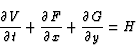(2.28)

where V=(uh,vh,h)t, F=(uuh+gh2/2,uvh,uh)t, G=(uvh,vvh+gh2/2,vh)t and H includes the Coriolis, dissipation and forcing terms. Therefore, there is no intuitive reason for lowering the order for one variable compared to the others. The only loss of similarity between these equations comes from the boundary conditions which only apply to the velocity. This is however a slight loss of similarity which only applies to the elements sharing a face or a vertex with the boundary. Hence, the need for lowering the order for pressure may not apply to all elements of the mesh. There is evidence, however, that it is better to use a combination of basis functions that fulfills the LBB condition, even in the broader context of the shallow water equations [Le Roux et al. , 1998]. Our own experience pinpoints that the behavior of the solution depends on the application. We are definitely missing a general theory of stability for the FE approximation in the broader context of the shallow water equations.

The fact that the pressure basis functions have to be of lower order compared to the velocity basis functions means that the overall truncation order of the stabilized FE methods for the shallow water equations is probably lower than the one permitted by the velocity basis functions. And, since the other disadvantage of these stable FE methods is the cumbersome and time consuming solving of a large matrix problem (especially when all the variables are coupled), it is doubtful that these methods can compete with todays FD ocean models in terms of cost and accuracy.

We focus herein on four different FE models: the Lynch and Werner model lynch87,lynch91 (also called the Quoddy model and the only one of the four used for coastal oceanography), the Le Roux et al. (2000; hereafter LLS) model, the Hua and Thomasset hua84 model and the Peraire et al. (1986; hereafter PZM) model. Only one model, the LLS model, among the four satisfies the LBB condition of stabilty. The Quoddy and the PZM models use a non-staggered (i.e., equal order) formulation of the variables and therefore require some kind of stabilizing trick'' which we will present and discuss. Due to their equal-order formulation, these two models are the simplest, in some sense, of the four for the same reason that the A-grid FD formulation is simpler than the other staggering techniques. In the context of the finite elements, there are some additional technical advantages to using non-staggering formulations which stems from a lower number of matrices to define and to inverse. Also, it unifies the use of gradient or divergence operators. In general, equal-order models are fairly easy to implement from scratch. Hence, they can be more appealing than more complex LBB complying formulations.

## The Galerkin FormulationMost FE methods are based on the Galerkin formulation. In these models, the domain,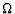, is broken up into a set of conformal elements (conformal in the sense that all elements connect to neighboring elements through common vertices). The form of the elements is rather unspecified but triangles or quadrangles are usually recommended. We favor the use of triangles (Fig. 2.5) because complex domains are more easily divided into triangles than quadrangles. For each vertex of the mesh, Mi, and in the context of linear finite elements, there is an associated basis function,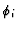. This basis function is piecewise linear in each triangle to which Mi belongs and forms a hat'' on top of Mi (Fig. 2.6). Over the rest of the domain, the basis function is zero. Let us consider the equation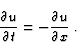(2.29)

u can be approximated by. The finite element approximation of this equation consists on multiplying 2.29 by a test function and then integrating the resulting equation over the whole domain. There is a certain freedom upon the choice for the test-function, though. In the collocation method, the test-function is defined as the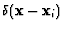(the Dirac-delta function). Then, the formulation bares similarities to the FD method. If both the basis functions and the test-functions are piecewise constant, the formulation is similar to the FV method. The Galerkin approximation is to take for the test-function,, which is used to approximate u. Thus, the discretized version of (2.29) is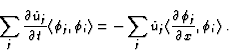(2.30)

where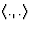is the inner product defined as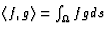. This is equivalent to say that the errors generated when discretizing (2.29) are projected onto another subspace of the function space (supposedly, a higher degree polynomial subspace). This formulation is said to be weak'' and is also referred to as the weighted residual approach. In some particular cases, it can be shown that the model equations can be described by a functional. This leads to the so-called variational principle. In such a case, the Galerkin approximation minimizes the approximation errors. Since the piecewise linear basis functions,, are not orthogonal, the terms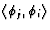lead to a matrix that has to be solved at each iteration in order to advance the solution in time. This matrix is usually referred to as the mass matrix and noted M. M is usually non-diagonal, but sparse. In order to gain computational efficiency, M is sometimes lumped''; that is, all non-diagonal terms are summed onto the diagonal to form an artificial diagonal mass matrix. This method bears similarities with the collocation method, as opposed to the Galerkin method, and can lead to a loss in accuracy.We now consider the issue of using irregularly spaced grid points in FD and FE methods. In the FD method, an irregular spacing of the nodes leads to a loss of order. Let us consider the equation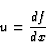(2.31)

Imagine three nodes located along one axis. The length between Node 1 and node 2 is A, and node 2 and node 3 are distanced by B. Without loss of generality, we can impose x1=-A, x2=0and x3=B (Fig. 2.7). The usual centered FD discretization of (2.31) at x2 gives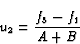(2.32)

As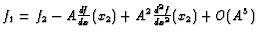and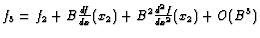,(2.33)

This formulation is second order if A=B but only first order if.

The same occurs for the FE method. Using linear hat'' functions to discretize this axis, the Galerkin discretization of (2.31) with the basis functions at node 2 as the test function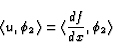(2.34)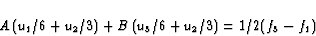(2.35)

where u and f are now approximated by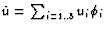and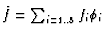. To demonstrate the problem of using irregular spacing, we use a different approach which consists of using polynomials of increasing order that satisfy (2.31). The maximum order for which (2.35) is consistent gives the truncation order of the scheme. If we take f(x)=1and u(x)=0, (2.35) is exactly satisfied. If we take f(x)=x and u(x)=1, the same applies. But if we take f(x)=x2 and u(x)=x, the equality is no longer true for the general case of, which means that the numerical method is only first order when the spacing is not constant. Therefore, we expect the order of any FE and FD method to be reduced in the presence of unstructured meshes.

## The Different Finite Element Models Tested

### The Quoddy Model

The Quoddy model of Lynch and Werner lynch87,lynch91 is a full 3D baroclinic finite element model. This model was successfully applied for coastal and tidal studies on the Scotian Shelf [Hannah et al. , 2000] and the Vancouver Island area [Foreman et al. , 2000]. It was modified to model the shallow water equations, retaining the main characteristic of the Quoddy model, which are: equal order of approximation for velocity and elevation, the divergence of the vertically integrated momentum equations can be recast by using the mass balance equation and eliminating the divergence of the vertically integrated mass flux. This yields a prognostic equation for the elevation of second order in time (a wave equation). Solving numerically for the three equations (two momentum equations and one wave equation) is easy and leads to a stable model, but, does not balance mass locally. To guaranty a better local conservation of mass, a weighted mass equation is added to the wave equation (the mass equation can also be viewed as a penalty term).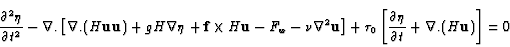(2.36)

The rational for mixing two equations that should be satisfied independently is that equal-order FE methods are usually unstable, the same way that the non-staggered A-grid is usually unstable for FD methods. Hence, the model is stabilized using physical principles (the divergence of the momentum equations) at the price that the local mass balance is not necessarily satisfied. This may have some influence on the dynamics of oceanic flows.

### The Peraire et al. (1986) Model

PZM developed an interesting model using equal-order interpolation and a two step explicit time-integration. First, mean values are computed for each triangle centroid from fluxes at vertices and then values at vertices are computed from flux computed at triangle centroids. This model is part of a broader family of Taylor-Galerkin formulations. We reproduce the demonstration about the Taylor-Galerkin formulation of Priestley pries92. Starting from the following prognostic equation in a conservation form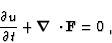(2.37)

the idea is to increase the accuracy of the finite differencing of the time operator by use of a Taylor's series: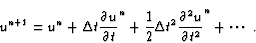(2.38)

By substituting the original equation (2.37) in the Taylor's series and truncating the series after the second order term yields(2.39)

The Galerkin formulation of this equation in a weak form is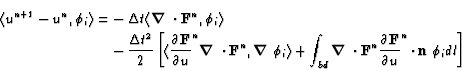(2.40)

where u and F are discretized using the piecewise linear basis functions. The difficulty at this stage is to express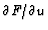. One way found by PZM was to approximateby a piecewise constant function and to express the one-step time integration as a two step time integration. Thus, if we first integrate forward in time over half a time step(2.41)

where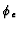is the piecewise constant basis function (one over one triangle and zero over the rest of the domain; the variables with the underscript e are approximated using these basis functions). The Taylor development of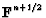at first order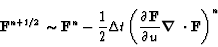(2.42)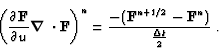(2.43)

Since the term on the left hand side is approximated using piecewise constant basis functions, (2.41) becomes(2.44)

In fact, we can integrate by part the first term on the right hand side in order to further simplify the equation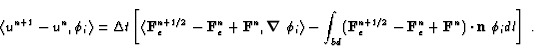(2.45)

Because of the use of linear basis function the first product in this equation can be further simplified to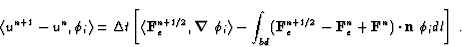(2.46)

This method presents some similarities with the Lax-Wendroff scheme. It is second order for smooth problems but might be over-dissipative at shocks. For purely advective problems, PZM found that this formulation behaves very well and we found that it outperforms the Quoddy model (not shown).

### The Hua and Thomasset (1984) Model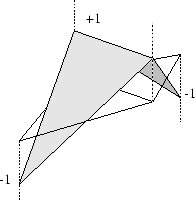Hua and Thomasset hua84 developed a finite element model staggered in space, using discontinuous linear non conforming (P1NC) basis functions for the velocity (Fig. 2.8) and the usual linear basis functions (P1) for the pressure. This formulation leads to a diagonal mass matrix for velocity, which leads to a simplified matrix problem to solve for the elevation when semi-implicitly discretizing in time. They claim the model to be oscillation-free, although LLS demonstrated that the Hua and Thomasset model does not satisfy the LBB condition of stability for the Stokes flow problem. In the shallow water context, it shows some signs of instability (not shown). After some tests, we chosed to integrate the equations explicitly in time using a Runge-Kutta integration technique instead of the semi-implicit technique proposed by Hua and Thomasset because the instability problem was then less severe.

### The Le Roux et al. (2000) Model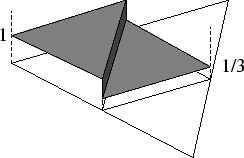LLS proposed to use a semi-implicit semi-Lagrangian time integration along with a spatial FE discretization that satisfies the LBB stability condition. The particularity of their choice for the basis functions resides in using macro-elements. Each macro-elements is cut into four sub-triangular elements. The basis functions for the velocity are linear inside each sub-triangle and the basis functions for the pressure (or elevation) are constant (see Fig. 2.9). The equation for the elevation can be inverted locally. Hence, the solution of the coupled system of shallow water equations can be reduced to solving Helmholtz-like coupled equations for the velocity components. In order to interpolate the variables at the previous time step on an unstructured mesh, they also proposed a high order kriging method (see Trochu, 1993, for a review). Using this interpolation technique, they found that the model was performing very well for the purely advective problem. However, the application to a finite element shallow water model was somewhat disappointing. The high order method destabilizes the model (personal com.). Therefore, a low order kriging method had to be used, leading to potentially high artificial viscosity. The mass was not conserved, forcing LLS to add a mass corrector. Another disadvantage of the LLS formulation is the fact that the elevation basis functions are piecewise constant. This means that the truncation order of the model for the elevation is lower than that for the velocity and might lower the order of the velocity as well, since the equations for velocities and elevation are coupled in the shallow water equations.Previous: Finite Difference Models
Next: The Discontinuous Spectral Element
Up: Presentation of the Numerical

Frederic Dupont
2001-09-11